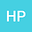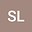Nodal solutions of fourth-order Kirchhoff equations with critical growth in $\R^N$
•••• Hongling Pu,
• Shiqi Li,
• Sihua Liang
Hongling Pu
Changchun Normal University

Corresponding Author:[email protected]

Author ProfileShiqi Li
Changchun Normal University
Author ProfileSihua Liang
Changchun Normal University
Author Profile## Abstract

In this paper, we consider the following fourth-order elliptic equations of Kirchhoff type with critical growth in $\R^N$: $$\Delta^2 u-\left(1+b\int_{\R^N} |\nabla u|^2 dx\right)\Delta u+V(x)u =\lambda f(u)+|u|^{2^{**}-2}u, \quad x\in\R^N,$$ where $\Delta^2 u$ is the biharmonic operator, $2^{**}=2N/(N-4)$ is the critical Sobolev exponent with $N\ge 5$, $b$ and $\lambda$ are two positive parameters, and $V(x)$ is the potential. By using a main tool of constrained minimization in Nehari manifold, we establish sufficient conditions for the existence result of nodal (that is, sign-changing) solutions.